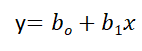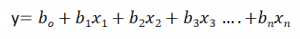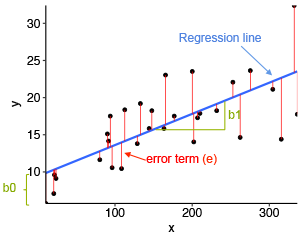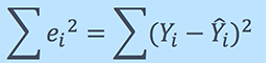This article was published as a part of the Data Science Blogathon

## Introduction

If you are reading this article, I am assuming that you are already into the Data Science world and have an idea about Machine Learning. If not, then no problem. I will start with the basic terminologies which one needs to know before understanding the main topic of discussion i.e. Linear Regression.

This article will cover everything you need to know about Linear Regression, the first Machine Learning algorithm of Data Science.

## Table of Content

1. Brief Introduction of Machine Learning and its types
2. Understanding Linear Regression
3. Assumptions of Linear Regression.
4. How to deal with the violation of Assumptions
5. Evaluation Metrics for Regression problems

## Introduction to Machine Learning

Machine learning is a branch of Artificial Intelligence (AI) focused on building applications that learn from data and improve their accuracy over time without being programmed to do so.

### Types of Machine Learning:

Supervised Machine Learning: It is an ML technique where models are trained on labeled data i.e output variable is provided in these types of problems. Here, the models find the mapping function to map input variables with the output variable or the labels.
Regression and Classification problems are a part of Supervised Machine Learning.

Unsupervised Machine Learning: It is the technique where models are not provided with the labeled data and they have to find the patterns and structure in the data to know about the data.
Clustering and Association algorithms are a part of Unsupervised ML.

## Understanding Linear Regression

In the most simple words, Linear Regression is the supervised Machine Learning model in which the model finds the best fit linear line between the independent and dependent variable i.e it finds the linear relationship between the dependent and independent variable.

Linear Regression is of two types: Simple and Multiple. Simple Linear Regression is where only one independent variable is present and the model has to find the linear relationship of it with the dependent variable

Whereas, In Multiple Linear Regression there are more than one independent variables for the model to find the relationship.

Equation of Simple Linear Regression, where bo is the intercept, b1 is coefficient or slope, x is the independent variable and y is the dependent variable.Equation of Multiple Linear Regressionwhere bo is the intercept, b1,b2,b3,b4…,bn are coefficients or slopes of the independent variables x1,x2,x3,x4…,xn and y is the dependent variable.A Linear Regression model’s main aim is to find the best fit linear line and the optimal values of intercept and coefficients such that the error is minimized.
Error is the difference between the actual value and Predicted value and the goal is to reduce this difference.

Let’s understand this with the help of a diagram.Image Source: Statistical tools for high-throughput data analysis

In the above diagram,

• x is our dependent variable which is plotted on the x-axis and y is the dependent variable which is plotted on the y-axis.
• Black dots are the data points i.e the actual values.
• bo is the intercept which is 10 and b1 is the slope of the x variable.
• The blue line is the best fit line predicted by the model i.e the predicted values lie on the blue line.

The vertical distance between the data point and the regression line is known as error or residual. Each data point has one residual and the sum of all the differences is known as the Sum of Residuals/Errors.

Mathematical Approach:

Residual/Error = Actual values – Predicted Values

Sum of Residuals/Errors = Sum(Actual- Predicted Values)

Square of Sum of Residuals/Errors = (Sum(Actual- Predicted Values))2

i.eFor an in-depth understanding of the Maths behind Linear Regression, please refer to the attached video explanation.

## Assumptions of Linear Regression

The basic assumptions of Linear Regression are as follows:

1. Linearity: It states that the dependent variable Y should be linearly related to independent variables. This assumption can be checked by plotting a scatter plot between both variables.

2. Normality: The X and Y variables should be normally distributed. Histograms, KDE plots, Q-Q plots can be used to check the Normality assumption.

Please refer to my attached blog for a detailed explanation on checking the normality and transforming the variables violating the assumption.

Source: https://heljves.com/gallery/vol_1_issue_1_2019_8.pdf

3. Homoscedasticity: The variance of the error terms should be constant i.e the spread of residuals should be constant for all values of X. This assumption can be checked by plotting a residual plot. If the assumption is violated then the points will form a funnel shape otherwise they will be constant.

Source: OriginLab

4. Independence/No Multicollinearity: The variables should be independent of each other i.e no correlation should be there between the independent variables. To check the assumption, we can use a correlation matrix or VIF score. If the VIF score is greater than 5 then the variables are highly correlated.

In the below image, a high correlation is present between x5 and x6 variables.

Source: towards data science

5. The error terms should be normally distributed. Q-Q plots and Histograms can be used to check the distribution of error terms.

Source: http://rstudio-pubs-static.s3.amazonaws.com

6. No Autocorrelation: The error terms should be independent of each other. Autocorrelation can be tested using the Durbin Watson test. The null hypothesis assumes that there is no autocorrelation. The value of the test lies between 0 to 4. If the value of the test is 2 then there is no autocorrelation.

Source: itfeature.com

## How to deal with the Violation of any of the Assumption

The Violation of the assumptions leads to a decrease in the accuracy of the model therefore the predictions are not accurate and error is also high.
For example, if the Independence assumption is violated then the relationship between the independent and dependent variable can not be determined precisely.

There are various methods are techniques available to deal with the violation of the assumptions. Let’s discuss some of them below.

Violation of Normality assumption of variables or error terms

To treat this problem, we can transform the variables to the normal distribution using various transformation functions such as log transformation, Reciprocal, or Box-Cox Transformation.
All the functions are discussed in this article of mine: How to transform into Normal Distribution

Violation of MultiCollineraity Assumption

It can be dealt with by:

• Doing nothing (if there is no major difference in the accuracy)
• Removing some of the highly correlated independent variables.
• Deriving a new feature by linearly combining the independent variables, such as adding them together or performing some mathematical operation.
• Performing an analysis designed for highly correlated variables, such as principal components analysis.

## Evaluation Metrics for Regression Analysis

To understand the performance of the Regression model performing model evaluation is necessary. Some of the Evaluation metrics used for Regression analysis are:

1. R squared or Coefficient of Determination: The most commonly used metric for model evaluation in regression analysis is R squared. It can be defined as a Ratio of variation to the Total Variation. The value of R squared lies between 0 to 1, the value closer to 1 the better the model.

where SSRES is the Residual Sum of squares and SSTOT is the Total Sum of squares

2. Adjusted R squared: It is the improvement to R squared. The problem/drawback with R2 is that as the features increase, the value of R2 also increases which gives the illusion of a good model. So the Adjusted R2 solves the drawback of R2. It only considers the features which are important for the model and shows the real improvement of the model.
Adjusted R2 is always lower than R2.

Source: stats.stackexchange.com

3. Mean Squared Error (MSE): Another Common metric for evaluation is Mean squared error which is the mean of the squared difference of actual vs predicted values.

Source: cppsecrets.com

4. Root Mean Squared Error (RMSE): It is the root of MSE i.e Root of the mean difference of Actual and Predicted values. RMSE penalizes the large errors whereas MSE doesn’t.

Source: community.qlik.com

## End Notes

We have covered most of the concepts of the Regression model in this blog. If you wish to explore more about the mathematics behind the model, please refer to the links attached to the blog.

Please feel free to connect with me on LinkedIn and share your valuable inputs. Kindly refer to my other articles here.

I am Deepanshi Dhingra currently working as a Data Science Researcher, and possess knowledge of Analytics, Exploratory Data Analysis, Machine Learning, and Deep Learning.

This article was published as a part of the Data Science Blogathon###### Deepanshi